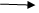Blog

Entity framework & Model class

21 September, 2018

Example to display the data in mvc with out using entity frame work(With out using model class)

Create a new mvc2 applicationAdd a new view page in home folder(sample)Go to home controller.csInclude the following the namespace

↓

Using system.data; Using system.data.sqlclient;

↓create the an action with name

↓write the following code   Public Action Result Sample () { Sql connection  con = new sql connection (“”); String s= “select * from emp details “; Sql data adapter da = new sql data adapter (s, con); Data set ds = new data set (); Da. Fill (ds, ‘x’); List <data row> r = new list <data row> (); For each (data row . x in ds. Tables .rows) R.add (); View data [“R”] = R; Return view (); }Go to sample viewWrite the following code <% @ Import  name space = ‘system.data’ % > <body> <h2>Satya technologies  </h2> <div> <% HTML Begin Form ();%> <table border = ‘2’ align = ‘center’ > <tr> <th>EMP id </th> <th> E NAME </th><th>E sal </th> </tr> <% for each (var x in (1 enumerable  <Data row> view data [‘R’])  I %)> <tr> <td><% : HTML. Encode (x %)></td> <td><% : HTML. Encode (x %)></td> <td><% : HTML. Encode (x %)></td> </tr> <% } %> </table> <% HTML. End form (); %> </div> </body>